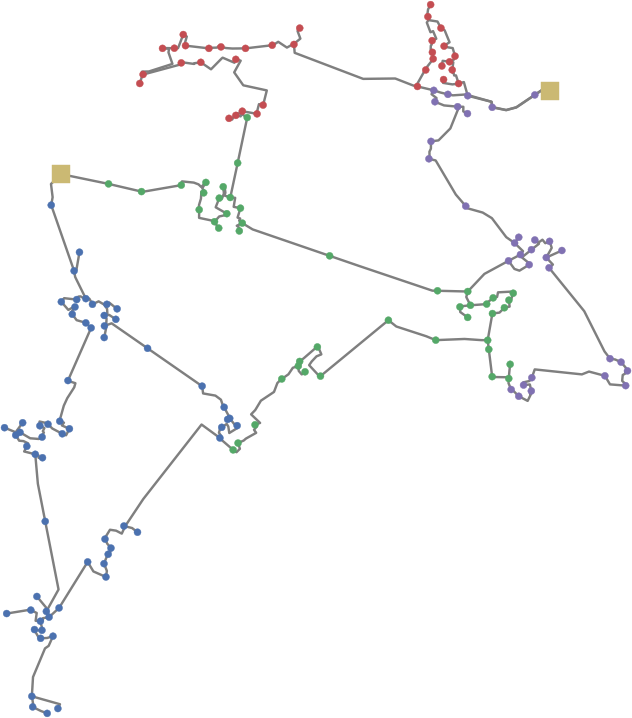# Draw Collections¶

pandapower.plotting.draw_collections(collections, figsize=(10, 8), ax=None, plot_colorbars=True, set_aspect=True, axes_visible=(False, False), copy_collections=True, draw=True)

Draws matplotlib collections which can be created with the create collection functions.

Input:
collections (list) - iterable of collection objects, may include tuples of collections
OPTIONAL:

figsize (tuple, (10,8)) - figsize of the matplotlib figure

ax (axis, None) - matplotlib axis object to plot into, new axis is created if None

plot_colorbars (bool, True) - defines whether colorbars should be plotted

set_aspect (bool, True) - defines whether ‘equal’ and ‘datalim’ aspects of axis scaling
should be set.

axes_visible (tuple, (False, False)) - defines visibility of (xaxis, yaxis)

OUTPUT:
ax - matplotlib axes

Example plot with mv_oberrhein network from the pandapower.networks package: